# T.R. Jain and V.K. Ohri Solutions Class 11 Statistics Economics Chapter 6 - Diagrammatic Presentation of Data- Bar Diagrams and Pies Diagrams

T.R. Jain and V.K. Ohri Solutions for Class 11 Statistics for Economics Chapter 6 – Diagrammatic Presentation of Data: Bar diagrams and Pie diagrams is regarded as an important concept to be studied thoroughly by the students. Here, we have provided T.R. Jain and V.K. Ohri Solutions for Class 11.

 Board CBSE Class Class 11 Subject Statistics for Economics Chapter Chapter 6 Chapter Name Diagrammatic Presentation of Data- Bar Diagrams and Pies Diagrams Number of questions solved 05 Category T.R. Jain and V.K. Ohri

Chapter 6: Diagrammatic Presentation of Data: Bar Diagrams and Pie Diagrams cover the below-mentioned concepts.

•       What is a bar diagram?
•       Types of bar diagram
•       Pie or Circular diagrams
•       Multiple bar diagram

## T.R. Jain and V.K. Ohri Solutions for Class 11 Statistics for Economics Chapter 6 – Diagrammatic Presentation of Data- Bar Diagrams and Pies Diagrams

Question 1

Represent the following data by a percentage bar diagram.

 Subjects Number of students 2016–17 2017–18 Statistics 25 30 Economics 40 42 History 35 28

Solution

 Subject 2016–17 2017–18 Number of students (%) Cumulative percentage Number of students (%) Cumulative percentage Statistics 25 25 30 30 Economics 40 60 42 72 History 35 100 28 100 Total 100 100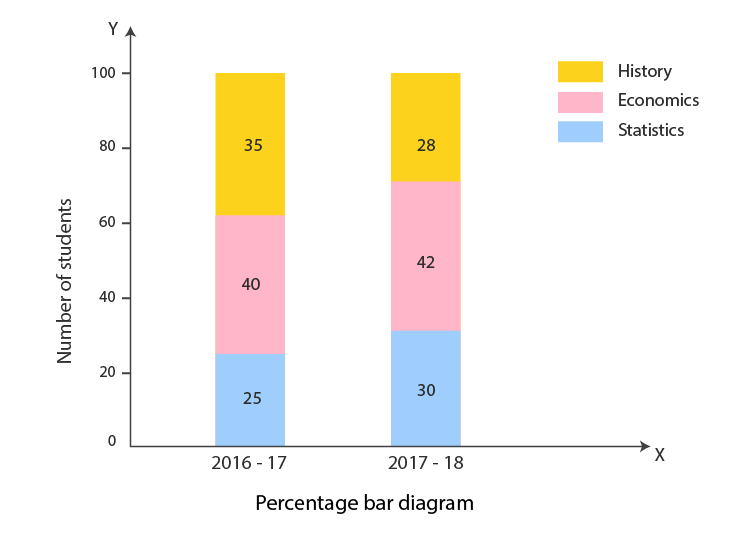Question 2

Draw a suitable diagram to represent the following information.

 Factory Selling price per unit (in ₹) Quantity sold Cost components (in ₹) Wages Materials Miscellaneous Total X 400 20 3,200 2,400 1,600 7,200 Y 600 30 6,000 6,000 9,000 21,000

Also, show profit and loss.

Solution

First of all, we shall calculate the cost (wages, materials, miscellaneous) and profit per unit as given in the following table.

 Factory X (20 units) Factory Y (30 units) Total cost (₹) Per unit cost (₹) Total cost (₹) Per unit cost (₹) Wages 3,200 160 6,000 200 Materials 2,400 120 6,000 200 Miscellaneous 1,600 80 9,000 300 Profit/Loss 800 (8,000–7,200) 40 -3,000 (18,000–21,000) -100

Note: (Negative profit is regarded as a loss.)

An appropriate diagram for representing this data would be the rectangle whose widths are in the ratio of the quantities sold, i.e, 20:30, i.e, 2:3. Selling prices would be represented by the corresponding heights of the rectangles with various costs (wages, materials, miscellaneous), and the profit or loss represented by the various divisions of the rectangles as shown in the diagram given on the next page.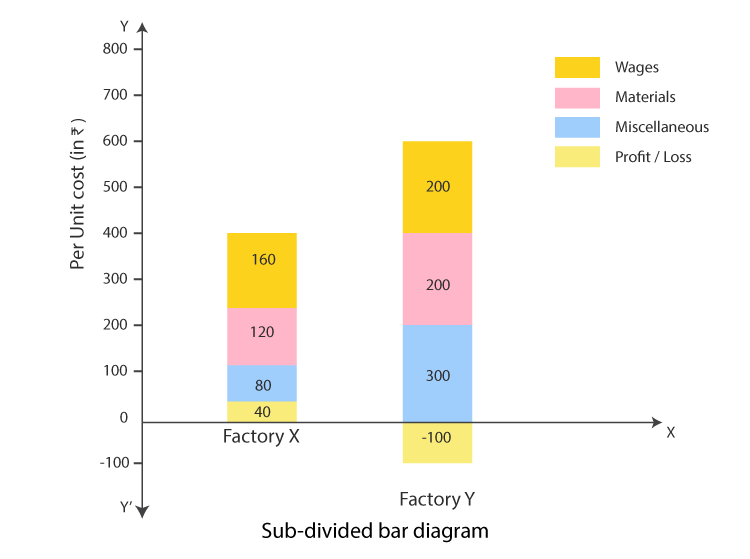(Note: In case of profit, i.e., when selling price > cost price, the entire rectangle will lie above the X-axis. However, in case of loss, we will have a rectangle with a portion lying below the X-axis which will reflect the loss incurred and it cannot be recovered through sales.)

Question 3

Following are the data about the market share of four brands of TV sets sold in Panipat and Ambala. Present the data in the pie chart.

 Brand of Sets Units sold in Panipat Units sold in Ambala Samsung 480 625 Akai 360 500 Onida 240 438 Sony 120 312

Solution

Total sets sold in Place A and Place B are 1,200 and 1,875 respectively. Data are to be represented by two circles whose radii are in the ratio of square roots of total TV sets sold in each city in the ratio of 1:1. The calculations regarding the construction of the pie diagram are as follows.

 Brands of Sets Place A Place B Sets sold Sales(₹) Sales in terms of components of 360° Sets sold Sales % Sales in terms of components of 360° Samsung 480 40 $$\begin{array}{l}\frac{40}{100}\, \times \, 360^{\circ}\, =\, 144^{\circ}\end{array}$$ 625 33.3 $$\begin{array}{l}\frac{33.3}{100}\, \times \, 360^{\circ}\, =\, 119.88^{\circ}\end{array}$$ Akai 360 30 $$\begin{array}{l}\frac{30}{100}\, \times \, 360^{\circ}\, =\, 108^{\circ}\end{array}$$ 500 26.7 $$\begin{array}{l}\frac{26.7}{100}\, \times \, 360^{\circ}\, =\, 96.12^{\circ}\end{array}$$ Onida 240 20 $$\begin{array}{l}\frac{20}{100}\, \times \, 360^{\circ}\, =\, 72^{\circ}\end{array}$$ 438 $$\begin{array}{l}\frac{23.4}{100}\, \times \, 360^{\circ}\, =\, 84.24^{\circ}\end{array}$$ Sony 120 10 $$\begin{array}{l}\frac{10}{100}\, \times \, 360^{\circ}\, =\, 36^{\circ}\end{array}$$ 312 16.6 $$\begin{array}{l}\frac{16.6}{100}\, \times \, 360^{\circ}\, =\, 59.76^{\circ}\end{array}$$ Total 1,200 360° 1,875 360°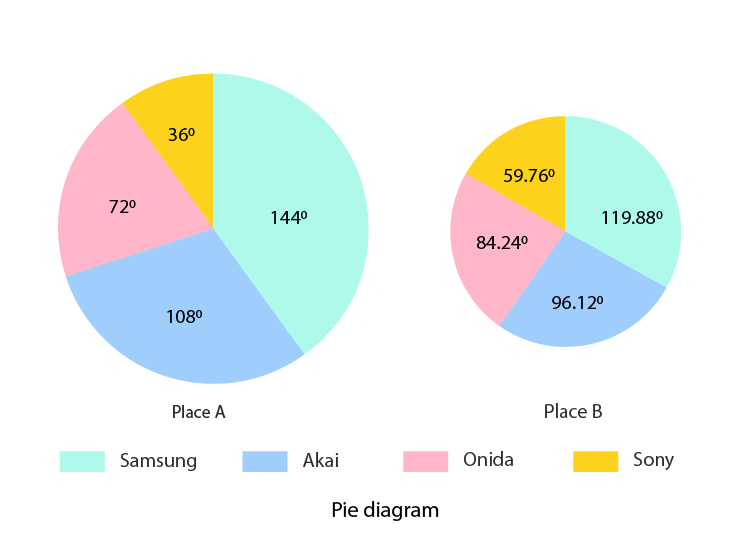Question 4

The following table shows the interest of students in a school in different games.

 Games Table Tennis Volleyball Hockey Basketball Cricket Number of Students 500 300 350 400 550

Solution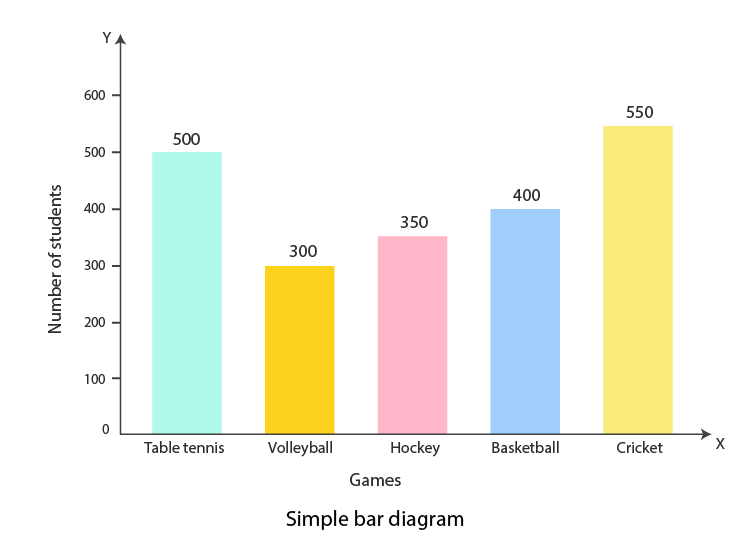Question 5

The following table shows the monthly expenditure of different families on different items.

 Items of expenditure Education Clothing Food Rent Other Total expenditure Family A 1,500 1,000 1,250 750 500 5,000 Family B 1,700 850 1,200 850 600 5,200 Family C 1,600 700 1,500 800 600 5,200

Represent the data in the form of a sub-divided bar diagram.

Solution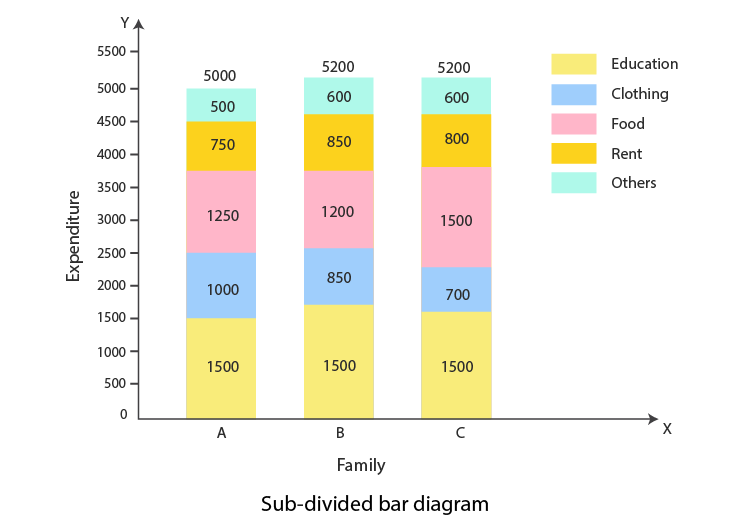Stay tuned to BYJU’S for more T.R. Jain and V.K. Ohri solutions, question papers, sample papers, syllabus and Commerce notifications.